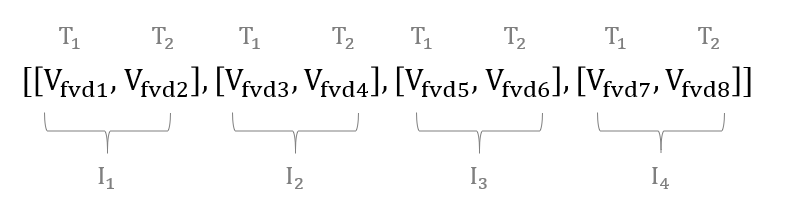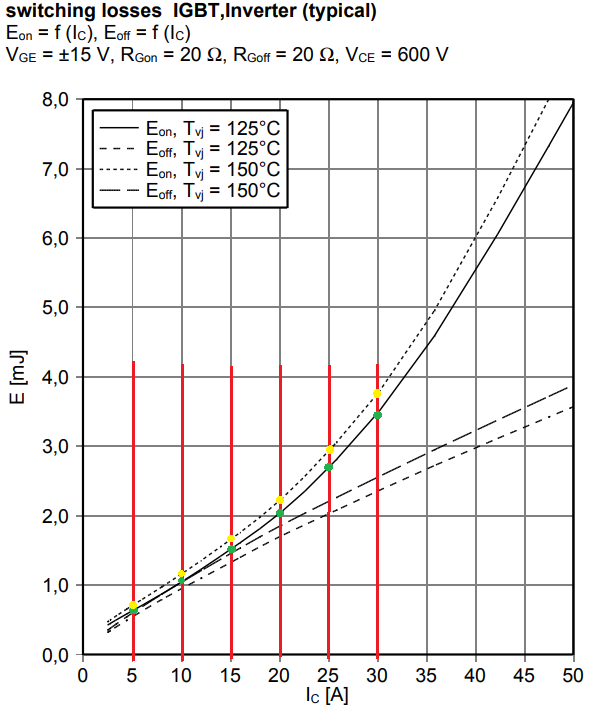# Importing power losses data

This section describes how to import power losses data from a manufacturer's datasheet or predefined XML files into the Typhoon HIL toolchain

## Import power losses data from XML files

Power losses data can be imported from standardized XML files using the XML buttons for the Switch element and for the Diode located on the component Mask. When activated, the element's corresponding property fields will be automatically filled with extracted data from the chosen XML files. Once the data is imported, changing the XML files will not affect the loaded data, unless files are imported again. Supported types of XML files are those that provide data for IGBT, MOSFET, and Diode switching elements.

The Typhoon HIL implementation of power loss data assumes the same current, voltage, and temperature vectors for all imported power losses tables. Sometimes the Switch (IGBT or MOSFET) and Diode vectors, can be slightly different because they are located in different files. When this is the case, the Switch vectors have priority for loss calculation. This means that any Diode vectors that are different will be substituted with vectors from the Switch element, and the Diode losses tables will be recalculated according to these newly assigned vectors. Of course, if converters or switching groups inside the converter consist of only Diode elements, vectors and tables from Diode XML files will be used without any additional changes or table recalculation.

## Import power losses data from datasheet

### Conduction loss parameters

Overall, conduction loss parameters can be specified in two ways: by using Look-up Tables or by supplying Voltage and resistance values (used for IGBTs). With the added support for MOSFET switching elements provided in the 2020.3, conduction losses can be specified only by using Lookup tables. In this case, forward voltage drop tables will be directly loaded from the .xml file to the Vt table and the Vd table. Conduction losses tables can also be extracted from the IGBT output characteristics and the Diode forward characteristics.

There are a few general rules for creating conduction loss Look-up Tables. When using 1D lookup tables, the current values are on the x-axis. An example of a general 1D lookup table for current values [I1, I2, I3, I4] is shown in Figure 1. This figure shows that the forward voltage drop Vfvd1 is related to current I1, Vfvd2 to current I2, and so on. An example of a general 2D lookup table for current values [I1, I2, I3, I4] and temperature values [T1, T2] is shown in Figure 2. In this case, the forward voltage drop Vfvd1 is related to current I1 and temperature T1; Vfvd2 is related to current I1 and temperature T2; Vfvd3 to current I2 and temperature T1; and so on.Figure 1. General 1D look up table for conduction lossesFigure 2. General 2D look up table for conduction lossesMOSFETs are bidirectional switching elements, so the Current values vector must consist of both positive and negative currents, for specifying the forward characteristic (Vt table) in both the first and third quadrant of operation. Because the same Current values vector is used for the inner body diode, which is a unidirectional switching element, the Vd table is defined based on the Current values sub-vector formed from the non-negative current values. For the IGBT case (unidirectional switch) the same Current values vector is used for the definition of the diode table (Vd table).

Before the 2020.3 Typhoon HIL Control Center release, conduction losses were only supported for IGBT switching elements and specified using calculated Voltage and resistance values. Conduction losses parameters can now be extracted from IGBT output characteristics and Diode forward characteristics.

In the following figures, characteristics are given for three temperatures (25℃, 125℃ and 150℃). It is important to first define the right temperature from which conduction loss parameters will be extracted. Usually this is the highest working temperature where the losses are maximized. The temperature dependance of conduction loss parameters is not modeled. Red lines represent the linear approximation of the given curves from the datasheet. The slope of these curves represent the resistance parameters (Rce for the IGBT and Rd for the Diode). The values for points where these curves cross the zero current are voltage parameters (Vce for IGBT and Vd for Diode).

Extracted parameters for IGBT conduction losses are:

${V}_{ce}=0.8V$

where points and are marked with green dots on IGBT_out.

Extracted parameters for Diode conduction losses are

${V}_{d}=0.75V$

Where points and are marked with green dots on Diode_fw.

### Switching losses parameters

Switching losses will be evaluated in the IGBT case for current values from 5 A to 30 A (marked with red lines in IGBT_turn_on, IGBT_turn_off, Diode_turn_off). Also, the zero current point will be added as a first value. It is important to have this initial zero point defined in order to avoid wrong results for small current values, because lookup tables extrapolate edge values. Switching characteristics are given only for one voltage value (Vce = 600 V). This means that 2D look up tables are sufficient for representing available data.

In this case, we use 2D lookup tables to describe the switching loss characteristics for different currents and temperatures. Field voltage values for this case should be left as default and as they won’t have an influence. The loss characteristics can also be given for different voltage values. When that is the case, 3D lookup tables should be used and voltage values that will influence the calculation of the switching losses should be included. In this case, there are two temperatures where the switching losses are given (125℃ and 150℃ ). Those values should be inserted in Temperature values fields.

IGBT_turn_on, IGBT_turn_off, Diode_turn_off represent switching losses characteristics extracted from component datasheet. Green dots represent values for 125℃ and yellow dots are for 150℃ . These values are used to fill the lookup table fields.

For the MOSFET case, where current (I) values can be negative, Switching Energy tables are defined based on the non-negative currents in the Current values vector.Figure 5. IGBT turn on losses characteristic

The extracted table for IGBT turn on losses is [[0, 0], [0.6e-3, 0.7e-3], [1.05e-3, 1.1e-3], [1.5e-3, 1.65e-3], [2e-3, 2.2e-3], [2.75e-3, 3e-3], [3.45e-3, 3.8e-3]].